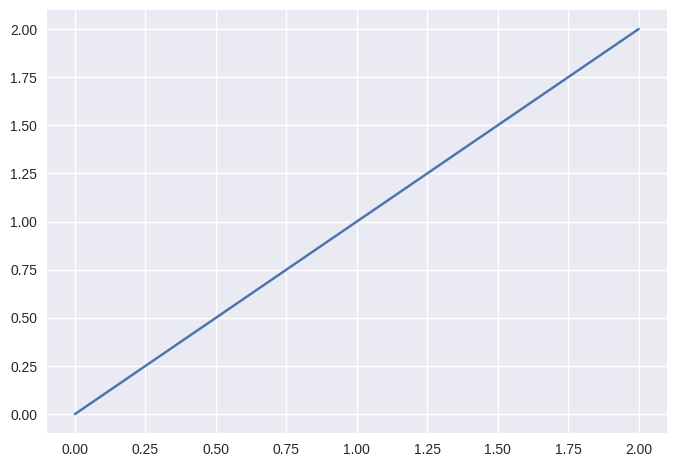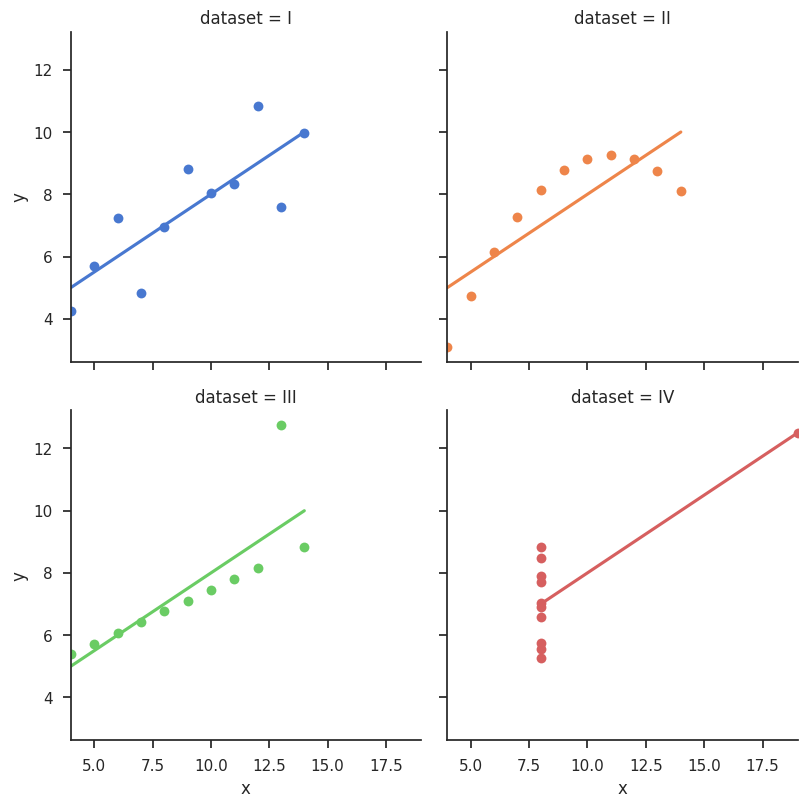settings:
show timestamps

# Ipynb test

Ipynb Summary
In :
```x = 2
y = 4
```

Code cell that should compute \$x^y\$:

In :
```print(x ** y)
```
```16
```

THIS CELL SHOULD BE HIDDEN

Some horrible code... (click to expand)
In :
```%matplotlib inline
print("Some horrible code is here")
```
```Some horrible code is here
```
In :
```import matplotlib.pyplot as plt
# plt.style.use('default')
plt.style.use('seaborn')
# hmm, classic has nice greyish background
# but seaborn uses more ticks?
import numpy as np
x = np.linspace(0, 2, 100)
plt.plot(x, x, label='linear')
plt.show()
```In :
```# demo from https://seaborn.pydata.org/examples/anscombes_quartet.html
#
import seaborn as sns
sns.set(style="ticks")

# Load the example dataset for Anscombe's quartet